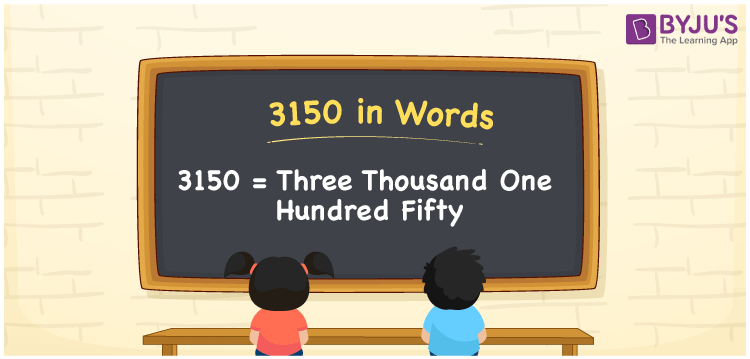# 3150 in Words

3150 in words is written as “Three Thousand One Hundred Fifty”. In Maths, 3150 is a cardinal number that expresses a quantity or a value. If the price of a mixer grinder is Rs.3150, then it can be represented as Rupees Three Thousand One Hundred Fifty only. Learn more about Numbers In Words at BYJU’S and how to spell 3150 in words, in this article.

 3150 in Words Three Thousand One Hundred Fifty Three Thousand One Hundred Fifty in Numbers 3150

## 3150 in English Words## How to Write 3150 in Words?

The number 3150 in words can be written using a place value method. Since 3150 is a four-digit number, hence, the place value of each digit is given by;

 Thousands Hundreds Tens Ones 3 1 5 0

From the above table,

3 → Thousands place

1 → Hundreds place

5 → Tens place

0 → Ones place

So, when we read the number from right to left, it is spelt as Three Thousand One Hundred Fifty.

### Expanded Form of 3150

We can write the expanded form as:

3 x Thousand + 1 × Hundred + 5 × Ten + 0 × One

= 3 x 1000 + 1 × 100 + 5 × 10 + 0 × 1

= 3000 + 100 + 50 + 0

= 3150

= Three Thousand One Hundred Fifty

3150 is a whole number that is succeeded by 3149 and preceded by 3151. Learn more about the number 3150 below:

• 3150 in Words – Three Thousand One Hundred Fifty
• Is 3150 an odd number? – No
• Is 3150 an even number? – Yes
• Is 3150 a perfect square number? – No
• Is 3150 a perfect cube number? – No
• Is 3150 a prime number? – No
• Is 3150 a composite number? – Yes

## Frequently Asked Questions on 3150 in words

Q1

### What is 3150 in words?

3150 in words is given by Three Thousand One Hundred Fifty.
Q2

### What is the rule to write 3150 in words?

3150 in words is written based on the place values of each digit. 3 is at thousands, 1 at hundreds, 5 at tens and 0 at ones. Thus, reading from right to left, it is written as Three Thousand One Hundred Fifty.
Q3

### What is the value of 3150 plus 200 in words?

3150 + 200 = 3350, i.e., Three Thousand Three Hundred Fifty in words